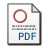# A Comment on “A Review of Student Test Properties in Condition of Multifactorial Linear Regression”

by Eisenstat, Eric
Published in Romanian Journal of Economic Forecasting
, 2010, volume 13 issue 3, 53-73Requires a PDF viewer such as Xpdf or Adobe Acrobat Reader  6733Kb

## Abstract

A recent article (Pavelescu, 2009) proposes a correction to the conventional student-t test of significance in linear regression models, but offers no formal description of its properties. This comment formally characterizes the sampling properties of the corrected student-t statistic. In application to multifactorial regressions, it turns out that the corrected student-t statistic is not ancillary – its sampling distribution depends on unknown nuisance parameters.Therefore, it is impossible to reasonably compute critical values and operatively designate a rejection criterion using such a test statistic, which makes the proposed testing procedure impractical. Some suggestions regarding the search for similar testing procedures are proposed and a Bayesian alternative is further discussed.

Keywords: multifactorial classical normal regression, collinearity, multicollinearity, significance test, sampling distributions, power functions, Bayesian linear regression, prior information, posterior distributions
JEL Classification:
C11, C12